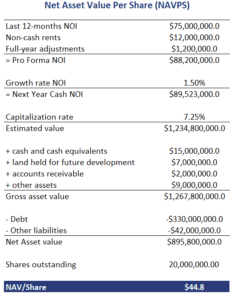# NAVPS Valuation

Net Asset Value Per Share (NAVPS) valuation is a valuation method for Real Estate Investment Trusts (REITs). It is often used by analysts because it can easily be compared to the price of the REIT’s stock. Thus, the NAVPS approach is an absolute valuation approach, although it can also be used for relative valuation. NAVPS is considered the best approach to value a REIT because it measures the fundamental value of a REIT.

On this page, we discuss how to calculate the NAVPS using accounting data. We also illustrate the calculation in Excel using an extensive example.

## NAVPS formula

NAVPS is the per share amount by which the assets of the REIT exceeds its liabilities. Importantly, we use market values rather than accounting book values. The first step in the approach is to calculate net operating income (NOI). For more information on how to calculate NOI, see our page on the direct capitalization Income Approach

It is important to use the expected NOI for next year. Also, we are interested in cash NOI. Thus, we should subtract all non-cash rent (average rent versus rent actually received). NOI is then capitalized using the capitalization rate. The cap rate is typically based on comparable recent property transactions.

Once we have capitalized NOI, we add the other assets and then subtract all liabilities. The result is net asset value of the REIT. Dividing by the number of shares we get the net asset value per share (NAVPS).

## NAVPS calculation example

Let’s have a look at a concrete example. Let’s consider an example of a retail REIT that needs to be valued. The following table illustrates all the necessary calculations needed to determine the net asset value per share.The resulting NAVPS can be compared to the REIT’s current stock price. If the price we obtain is above the NAVPS, the stocks is undervalued. If the price is below the actual stock price, the REIT is overvalued. Finally, if the price is the same, the NAVPS valuation suggests the REIT is fairly valued.

## Summary

We discussed the NAVPS valuation approach. This approach is the most commonly used approach to value a REIT. That’s because it provides an analyst with the fundamental value of the company.

### NAVPS calculator

Want to have an implementation in Excel? Download the Excel file: NAVPS Valuation